Next: Laws of Thermodynamics Up: Statistical Thermodynamics Previous: Uses of Entropy

# Entropy and Quantum Mechanics

The entropy,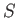, of a system is defined in terms of the number,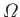, of accessible microstates consistent with an overall energy in the range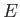to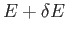via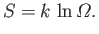(5.92)

We have already demonstrated that this definition is utterly insensitive to the resolution,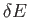, to which the macroscopic energy is measured (See Section 5.7.) In classical mechanics, if a system possesses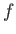degrees of freedom then phase-space is conventionally subdivided into cells of arbitrarily chosen volume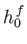. (See Section 3.2.) The number of accessible microstates is equivalent to the number of these cells in the volume of phase-space consistent with an overall energy of the system lying in the rangeto. Thus,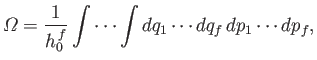(5.93)

giving(5.94)

Thus, in classical mechanics, the entropy is undetermined to an arbitrary additive constant that depends on the size of the cells in phase-space. In fact,increases as the cell size decreases. The second law of thermodynamics is only concerned with changes in entropy, and is, therefore, unaffected by an additive constant. Likewise, macroscopic thermodynamical quantities, such as the temperature and pressure, that can be expressed as partial derivatives of the entropy with respect to various macroscopic parameters [see Equations (5.85) and (5.86)] are unaffected by such a constant. So, in classical mechanics, the entropy is rather like a gravitational potential--it is undetermined to an additive constant, but this does not affect any physical laws.

The non-unique value of the entropy comes about because there is no limit to the precision to which the state of a classical system can be specified. In other words, the cell size,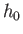, can be made arbitrarily small, which corresponds to specifying the particle coordinates and momenta to arbitrary accuracy. However, in quantum mechanics, the uncertainty principle sets a definite limit to how accurately the particle coordinates and momenta can be specified. (See Section C.8.) In general,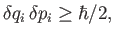(5.95)

where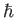is Planck's constant over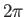,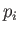is the momentum conjugate to the generalized coordinate, and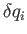,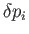are the uncertainties in these quantities, respectively. In fact, in quantum mechanics, the number of accessible quantum states with the overall energy in the rangetois completely determined. This implies that, in reality, the entropy of a system has a unique and unambiguous value. Quantum mechanics can often be mocked up'' in classical mechanics by setting the cell size in phase-space equal to Planck's constant, so that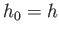. This automatically enforces the most restrictive form of the uncertainty principle,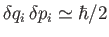. In many systems, the substitution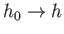in Equation (5.94) gives the same, unique value foras that obtained from a full quantum-mechanical calculation. (See Section 8.10.)

Consider a simple quantum-mechanical system consisting of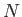non-interacting spinless particles of mass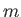confined in a cubic box of dimension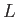. (See Section C.10.) The energy levels of the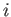th particle are given by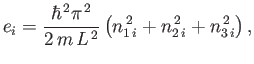(5.96)

where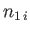,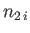, and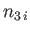are three (positive) quantum numbers. The overall energy of the system is the sum of the energies of the individual particles, so that for a general state,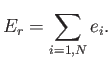(5.97)

The overall state of the system is completely specified by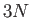quantum numbers, so the number of degrees of freedom is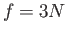. The classical limit corresponds to the situation where all of the quantum numbers are much greater than unity. In this limit, the number of accessible states varies with energy according to our usual estimate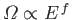. (See Section 3.8.) The lowest possible energy state of the system, the so-called ground-state, corresponds to the situation where all quantum numbers take their lowest possible value, unity. Thus, the ground-state energy,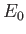, is given by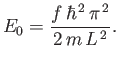(5.98)

There is only one accessible microstate at the ground-state energy (i.e., that where all quantum numbers are unity), so by our usual definition of entropy,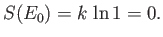(5.99)

In other words, there is no disorder in the system when all the particles are in their ground-states.

Clearly, as the energy approaches the ground-state energy, the number of accessible states becomes far less than the usual classical estimate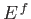. This is true for all quantum mechanical systems. In general, the number of microstates varies roughly like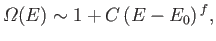(5.100)

where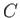is a positive constant. According to Equation (5.31), the temperature varies approximately like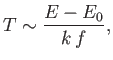(5.101)

provided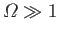. Thus, as the absolute temperature of a system approaches zero, the internal energy approaches a limiting value(the quantum-mechanical ground-state energy), and the entropy approaches the limiting value zero. This proposition is known as the third law of thermodynamics.

At low temperatures, great care must be taken to ensure that equilibrium thermodynamical arguments are applicable, because the rate of attaining equilibrium may be very slow. Another difficulty arises when dealing with a system in which the atoms possess nuclear spins. Typically, when such a system is brought to a very low temperature, the entropy associated with the degrees of freedom not involving nuclear spins becomes negligible. Nevertheless, the number of microstates,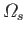, corresponding to the possible nuclear spin orientations may be very large. Indeed, it may be just as large as the number of states at room temperature. The reason for this is that nuclear magnetic moments are extremely small, and, therefore, have extremely weak mutual interactions. Thus, it only takes a tiny amount of heat energy in the system to completely randomize the spin orientations. Typically, a temperature as small as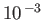degrees kelvin is sufficient to randomize the spins.

Suppose that the system consists ofatoms with nuclei of spin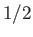. Each spin can have two possible orientations. If there is enough residual heat energy in the system to randomize the spins then each orientation is equally likely. It follows that there are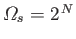accessible spin states. The entropy associated with these states is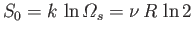. Below some critical temperature,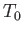, the interaction between the nuclear spins becomes significant, and the system settles down in some unique quantum-mechanical ground-state (e.g., with all spins aligned). In this situation,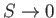, in accordance with the third law of thermodynamics. However, for temperatures which are small, but not small enough to freeze out'' the nuclear spin degrees of freedom, the entropy approaches a limiting value,, that depends only on the kinds of atomic nuclei in the system. This limiting value is independent of the spatial arrangement of the atoms, or the interactions between them. Thus, for most practical purposes, the third law of thermodynamics can be written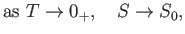(5.102)

where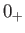denotes a temperature that is very close to absolute zero, but still much larger than. This modification of the third law is useful because it can be applied at temperatures that are not prohibitively low.Next: Laws of Thermodynamics Up: Statistical Thermodynamics Previous: Uses of Entropy
Richard Fitzpatrick 2016-01-25